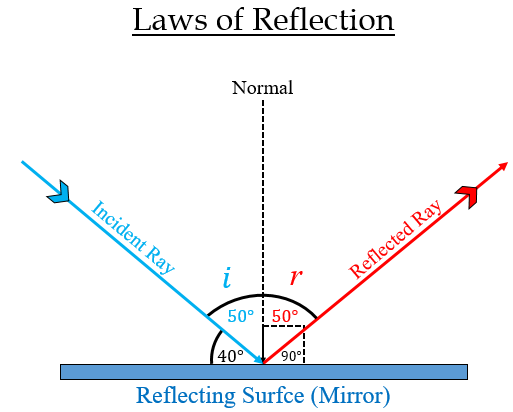# A ray of light strikes a plane mirror at an angle of 40° to the mirror surface. What will be the angle of reflection?

Here, the angle to the mirror surface (the angle between the incident ray and the mirror) = 40°

Therefore, the angle of incidence (the angle between the incident ray and the normal) = 90°$-$ 40°= 50°

Now, we know that according to the law of reflection, the angle of incidence is equal to the angle of reflection.

Therefore, the angle of reflection will be 50°.

Image is posted for reference only.Updated on: 10-Oct-2022

3K+ Views